andrea.negrete

•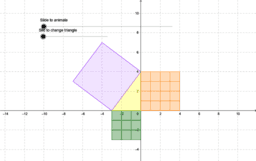Pythagorean Theorem Proof

Activity

andrea.negrete

•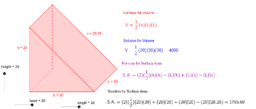Right Triangular Prism 2

Activity

andrea.negrete

•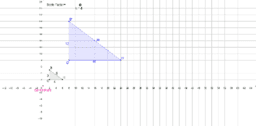Enlargement with scale factor

Activity

andrea.negrete

•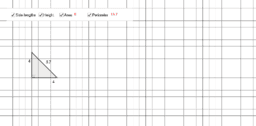Triangle Area and Perimeter

Activity

andrea.negrete

•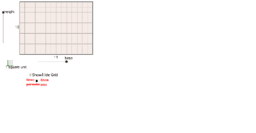Area and Perimeter of Rectangles

Activity

andrea.negrete

•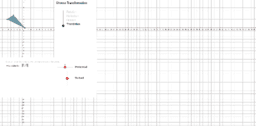Transformations

Activity

andrea.negrete

•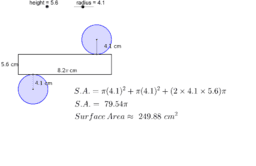Cylinder - Volume and Surface Area

Activity

andrea.negrete

•Triangular Prism - Surface Area and Volume

Activity

andrea.negrete

•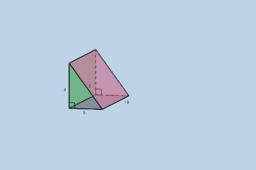Triangular Prism - Surface Area and Volume

Activity

andrea.negrete

•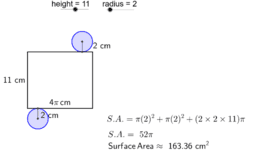Cylinder - Surface Area and Volume

Activity

andrea.negrete

•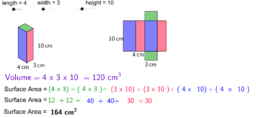Rectangular Prism - Surface Area and Volume

Activity

andrea.negrete

•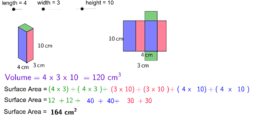Rectangular Prisms - Surface Area and Volume

Activity

andrea.negrete

•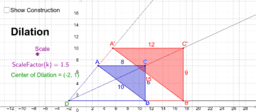Dilation Demonstration

Activity

andrea.negrete

•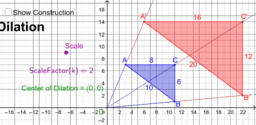Dilation Demonstration

Activity

andrea.negrete

•Rotation around a point

Activity

andrea.negrete